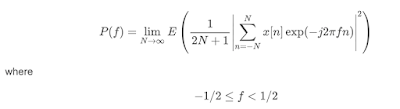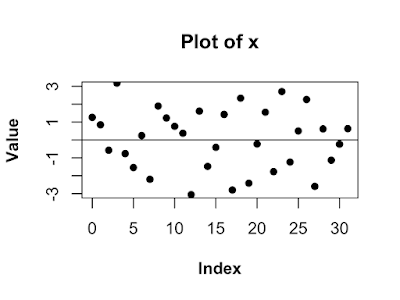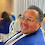## Thursday, June 13, 2019

### Periodogram with R

Periodogram with R
The power spectral density (PSD) is a function that describes the distribution of power over the frequency components composing our data set. If we knew the process that generated the data, we could just calculate the PSD; we would not have to estimate it. Unfortunately, in practice we won't have access to the random process, only the samples (data) produced by the process. So, we can't get the true PSD, we can only get an estimate of the true PSD. In our example below, we made the data, so we know what the true PSD should look like.
The PSD is written aswhere -1/2 <= f < 1/2 x is the data, e is the complex exponential, the sum of the data x multiplied by e is the Fourier transform. In English, calculate the PSD by finding the expected value of the magnitude squared of the Fourier transform as the amount of data increases to infinity. Well, that's a mouthful! The frequency f is expressed in normalized frequency. Normalized frequency is the frequency divided by the sampling rate. Normalized frequency is often used for simplicity. To convert to frequency in Hz multiply by the sampling rate.
The periodogram estimator is based on the definition in equation above. The definition has a limit and an expected value. To use the definition directly we would need an infinite amount of data, which we don't have, and we would need to know the probability density function (PDF) of the data which we don't know. To use this definition to get an estimator of the PSD, we must drop the limit and we must drop the expectation operator (\$E\$). We also can only evaluate a finite number of frequencies. After dropping the limit and the expectation we are left withwhere 0 <= f < 1 and N is the number of data samples. Notice, the range of f changes from (-1/2,1/2) in the first equation to (0,1) in the second equation. Textbooks usually use the former and R uses the latter.
The periodogram is very easy to implement in R, but before we do we need to simulate some data. The code below first uses the set.seed command so R will produce the same "random" numbers each time. Then it creates a 32 normally distributed numbers and 32 points of a sine wave with a normalized frequency of 0.4 and a amplitude of 2. The signal is made up of a sine wave and the random points added together.
The figure below is a plot of the data generated above. To me it looks random and it is not obvious there is a sine wave in there.
```set.seed(0)
N <-32
n<- 0:(N-1)
w <- rnorm(1:N)
f1 <- 0.4
A1 <- 2
s <- A1*sin(2*pi*f1*n)
x <- s + w
```
Now we are going to implement the periodogram in R. It is pretty much a direct translation from the math. We start with the 1/N and multiply that by the absolute value of the square of the Fourier transform of the data. Writing that in English was more work than implementing the periodogram in R!```xPer <- (1/N)*abs(fft(x)^2)
f    <- seq(0,1.0-1/N,by=1/N)
```
The figure below is a plot of the periodogram of the data. The dotted line marks the location of the frequency f1, the frequency of the sine wave of the data. Now the sine wave component really stands out!. Notice two things. First, the peaks of the periodogram seems to be a bit off the true values. Second, the plot looks jagged. Both of these things are caused by the same thing: the periodogram is only evaluated at 32 frequency bins. The frequencies we evaluate the periodogram on are called bins. We can fix both problems by evaluating the periodogram at more bins. One way to evaluate the periodogram at more points is to get more data! That would certainly fix both problems. Unfortunately, we often are struck with the data we have. There is another way.Since f is a continuous variable we can evaluate the periodogram at as many points as we want. The way we do this is to pretend we have more data by sticking zeros at the end of our data, we zero pad it. The R code below adds 968 zeros to the end of x zero-padding it to a total of 1000 "data" points. As you can see in the figure below, we have fixed both problems. In the 32 point periodogram missed the peak, because the periodogram was not evaluated at enough points. The zero-padding does not guarantee we hit the peak, but it will get closer. Also, we fixed the jaggedness by evaluating the periodogram at many more bins.
```xzp <- c(x,rep(0,1000-N))
Nzp <- length(xzp)
```
```xPerZp <- (1/N)*abs(fft(xzp)^2)
fzp    <- seq(0,1.0-1/Nzp,by=1/Nzp)
```#### 1 comment:

1.